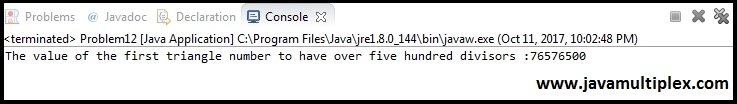## Problem Description:

The sequence of triangle numbers is generated by adding the natural numbers. So the 7th triangle number would be 1 + 2 + 3 + 4 + 5 + 6 + 7 = 28. The first ten terms would be:

1, 3, 6, 10, 15, 21, 28, 36, 45, 55, ...

Let us list the factors of the first seven triangle numbers:
1: 1
3: 1,3
6: 1,2,3,6
10: 1,2,5,10
15: 1,3,5,15
21: 1,3,7,21
28: 1,2,4,7,14,28

We can see that 28 is the first triangle number to have over five divisors.

What is the value of the first triangle number to have over five hundred divisors?Figure1

## Concept:

If you know how to find triangular number and divisors of given number then you can easily solve this problem. Use below steps to solve it.
1. Find triangular number.
2. Find number of divisors of triangular number that we got in step 1.
3. If number of divisors are greater than 500, print triangular number otherwise continue.

## Output:Figure2

## References:

Thank you friends, I hope you have clearly understood the solution of this problem. If you have any doubt, suggestion or query please feel free to comment below. You can also discuss this solution in our forum.Project Euler | Problem 12 | Highly divisible triangular numberReviewed by Rohit Agarwal on 10/11/2017 Rating: 5